NetworkX

transitivity

#### Next topic

average_clustering

# clustering¶

clustering(G, nodes=None, weight=None)[source]

Compute the clustering coefficient for nodes.

For unweighted graphs, the clustering of a node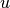is the fraction of possible triangles through that node that exist,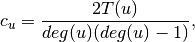where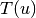is the number of triangles through nodeand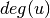is the degree of.

For weighted graphs, the clustering is defined as the geometric average of the subgraph edge weights [R185],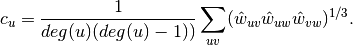The edge weights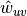are normalized by the maximum weight in the network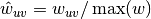.

The value of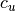is assigned to 0 if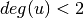.

Parameters : G : graph nodes : container of nodes, optional (default=all nodes in G) Compute clustering for nodes in this container. weight : string or None, optional (default=None) The edge attribute that holds the numerical value used as a weight. If None, then each edge has weight 1. out : float, or dictionary Clustering coefficient at specified nodes

Notes

Self loops are ignored.

References

 [R185] (1, 2) Generalizations of the clustering coefficient to weighted complex networks by J. Saramäki, M. Kivelä, J.-P. Onnela, K. Kaski, and J. Kertész, Physical Review E, 75 027105 (2007). http://jponnela.com/web_documents/a9.pdf

Examples

>>> G=nx.complete_graph(5)
>>> print(nx.clustering(G,0))
1.0
>>> print(nx.clustering(G))
{0: 1.0, 1: 1.0, 2: 1.0, 3: 1.0, 4: 1.0}